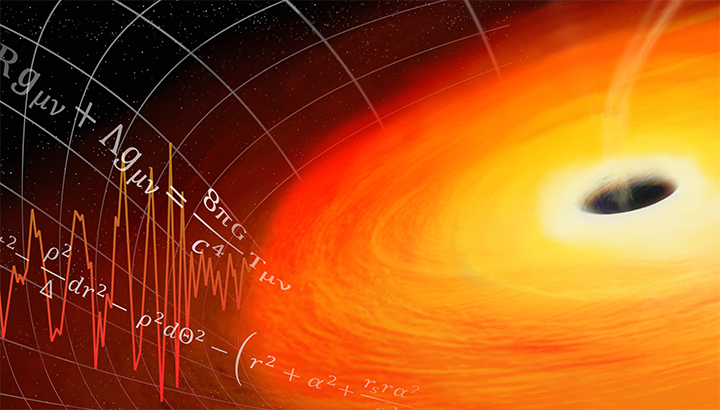The equations of general relativity, Einstein's field equations, are among the most complicated partial differential equations in mathematical physics. These equations predict the existence of gravitational waves, which are propagating disturbances in spacetime itself. In 2016, the first direct observation of these waves from colliding black holes was reported on, a historic discovery that led to last years Nobel Prize. This discovery would not have been possible without intense interaction between physicists, mathematicians, and high-performance computing tools. Indeed, numerically solving Einstein's equations for the expected wave signal and the processing of gravitational-wave datasets was enabled by advances in algorithms, numerical methods, and access to large computing resources. In this talk, I will focus on the critical role all three played in making this historic discovery as well as summarizing current directions in computational relativity and gravitational-wave data science.